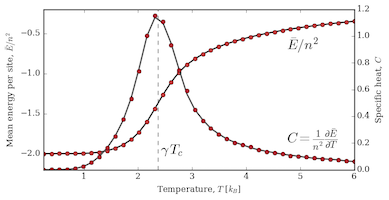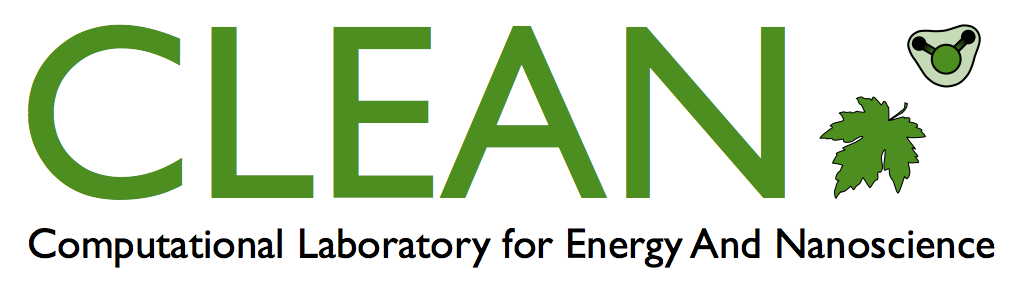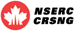Computational Laboratory for Energy And NanoscienceManuscript Summary - Deep neural networks for learning operators through observation: the case of the 2d Ising modelIn this article, we show that deep neural networks (CNN) are able to learn how to compute properties of physical models through observation alone. You do not need to "teach" them equations or math. Here, we showed a CNN lots of examples of checkboard patterns, where each had a simple energy based on a "white likes white, black likes black" model. This is the same model we explored here, except now we used deep learning rather than conventational machine learning approaches. The deep learning model we trained is so good that it can be used to explore phase transitions. We also show that it is really efficient. For a more difficult energy (one based on charged particles which have long range interactions), we trained a DNN that was 3 orders of magnitude (i.e. 1000x) faster than a conventional calculation. https://journals.aps.org/pre/abstract/10.1103/PhysRevE.97.032119